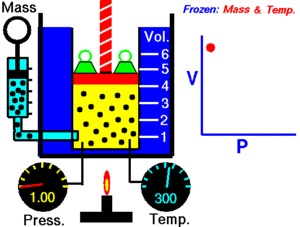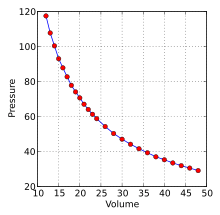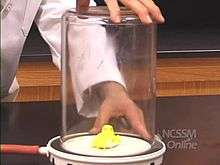# Boyle's lawAn animation showing the relationship between pressure and volume when amount and temperature are held constant.

Boyle's law (sometimes referred to as the Boyle–Mariotte law, or Mariotte's law) is an experimental gas law that describes how the pressure of a gas tends to increase as the volume of the container decreases. A modern statement of Boyle's law is

The absolute pressure exerted by a given mass of an ideal gas is inversely proportional to the volume it occupies if the temperature and amount of gas remain unchanged within a closed system.

Mathematically, Boyle's law can be stated asorwhere P is the pressure of the gas, V is the volume of the gas, and k is a constant.

The equation states that product of pressure and volume is a constant for a given mass of confined gas as long as the temperature is constant. For comparing the same substance under two different sets of condition, the law can be usefully expressed asThe equation shows that, as volume increases, the pressure of the gas decreases in proportion. Similarly, as volume decreases, the pressure of the gas increases. The law was named after chemist and physicist Robert Boyle, who published the original law in 1662.

## HistoryA graph of Boyle's original data

This relationship between pressure and volume was first noted by Richard Towneley and Henry Power. Robert Boyle confirmed their discovery through experiments and published the results. According to Robert Gunther and other authorities, it was Boyle's assistant, Robert Hooke, who built the experimental apparatus. Boyle's law is based on experiments with air, which he considered to be a fluid of particles at rest in between small invisible springs. At that time, air was still seen as one of the four elements, but Boyle disagreed. Boyle's interest was probably to understand air as an essential element of life; for example, he published works on the growth of plants without air. Boyle used a closed J-shaped tube and after pouring mercury from one side he forced the air on the other side to contract under the pressure of mercury. After repeating the experiment several times and using different amounts of mercury he found that under controlled conditions, the pressure of a gas is inversely proportional to the volume occupied by it. The French physicist Edme Mariotte (1620–1684) discovered the same law independent of Boyle in 1679, but Boyle had already published it in 1662. Thus this law is sometimes referred to as Mariotte's law or the Boyle–Mariotte law. Later, in 1687 in the Philosophiæ Naturalis Principia Mathematica, Newton showed mathematically that if an elastic fluid consisting of particles at rest, between which are repulsive forces inversely proportional to their distance, the density would be directly proportional to the pressure, but this mathematical treatise is not the physical explanation for the observed relationship. Instead of a static theory a kinetic theory is needed, which was provided two centuries later by Maxwell and Boltzmann.

This law was the first physical law to be expressed in the form of an equation describing the dependence of two variable quantities.

## DefinitionBoyle's law demonstrations

The law itself can be stated as follows:

For a fixed amount of an ideal gas kept at a fixed temperature, pressure and volume are inversely proportional.

Or Boyle's law is a gas law, stating that the pressure and volume of a gas have an inverse relationship, when temperature is held constant. If volume increases, then pressure decreases and vice versa, when temperature is held constant.

Therefore, when the volume is halved, the pressure is doubled; and if the volume is doubled, the pressure is halved.

### Relation with kinetic theory and ideal gases

Boyle's law states that at constant temperature for a fixed mass, the absolute pressure and the volume of a gas are inversely proportional. The law can also be stated in a slightly different manner, that the product of absolute pressure and volume is always constant.

Most gases behave like ideal gases at moderate pressures and temperatures. The technology of the 17th century could not produce high pressures or low temperatures. Hence, the law was not likely to have deviations at the time of publication. As improvements in technology permitted higher pressures and lower temperatures, deviations from the ideal gas behavior became noticeable, and the relationship between pressure and volume can only be accurately described employing real gas theory. The deviation is expressed as the compressibility factor.

Boyle (and Mariotte) derived the law solely on experimental grounds. The law can also be derived theoretically based on the presumed existence of atoms and molecules and assumptions about motion and perfectly elastic collisions (see kinetic theory of gases). These assumptions were met with enormous resistance in the positivist scientific community at the time however, as they were seen as purely theoretical constructs for which there was not the slightest observational evidence.

Daniel Bernoulli in 1737-1738 derived Boyle's law using Newton's laws of motion with application on a molecular level. It remained ignored until around 1845, when John Waterston published a paper building the main precepts of kinetic theory; this was rejected by the Royal Society of England. Later works of James Prescott Joule, Rudolf Clausius and in particular Ludwig Boltzmann firmly established the kinetic theory of gases and brought attention to both the theories of Bernoulli and Waterston.

The debate between proponents of Energetics and Atomism led Boltzmann to write a book in 1898, which endured criticism up to his suicide in 1906. Albert Einstein in 1905 showed how kinetic theory applies to the Brownian motion of a fluid-suspended particle, which was confirmed in 1908 by Jean Perrin.

### Equation

The mathematical equation for Boyle's law is:where:

• P denotes the pressure of the system.
• V denotes the volume of the gas.
• z is a constant value representative of the pressure and volume of the system.

So long as temperature remains constant the same amount of energy given to the system persists throughout its operation and therefore, theoretically, the value of k will remain constant. However, due to the derivation of pressure as perpendicular applied force and the probabilistic likelihood of collisions with other particles through collision theory, the application of force to a surface may not be infinitely constant for such values of v, but will have a limit when differentiating such values over a given time. Forcing the volume V of the fixed quantity of gas to increase, keeping the gas at the initially measured temperature, the pressure p must decrease proportionally. Conversely, reducing the volume of the gas increases the pressure. Boyle's law is used to predict the result of introducing a change, in volume and pressure only, to the initial state of a fixed quantity of gas.

The initial and final volumes and pressures of the fixed amount of gas, where the initial and final temperatures are the same (heating or cooling will be required to meet this condition), are related by the equation:Here P1 and V1 represent the original pressure and volume, respectively, and P2 and V2 represent the second pressure and volume.

Boyle's law, Charles's law, and Gay-Lussac's law form the combined gas law. The three gas laws in combination with Avogadro's law can be generalized by the ideal gas law.

## Boyle's law and the human breathing system

Boyle's law is often used as part of an explanation on how the breathing system works in the human body. This commonly involves explaining how the lung volume may be increased or decreased and thereby cause a relatively lower or higher air pressure within them (in keeping with Boyle's law). This forms a pressure difference between the air inside the lungs and the environmental air pressure, which in turn precipitates either inhalation or exhalation as air moves from high to low pressure.

## Citations

1. Draper, John William (1861). A Textbook on chemistry. p. 46.
2. Levine, Ira. N (1978). "Physical Chemistry" University of Brooklyn: McGraw-Hill
3. Levine, Ira. N. (1978), p12 gives the original definition.
5. See:
• Henry Power, Experimental Philosophy, in Three Books … (London, England: Printed by T. Roycroft for John Martin and James Allestry, 1663), pp. 126-130. Available on-line at: Early English Books Online. On page 130, Power presents (not very clearly) the relation between the pressure and the volume of a given quantity of air: "That the measure of the Mercurial Standard, and Mercurial Complement, are measured onely by their perpendicular heights, over the Surface of the restagnant Quicksilver in the Vessel: But Ayr, the Ayr's Dilatation, and Ayr Dilated, by the Spaces they fill. So that here is now four Proportionals, and by any three given, you may strike out the fourth, by Conversion, Transposition, and Division of them. So that by these Analogies you may prognosticate the effects, which follow in all Mercurial Experiments, and predemonstrate them, by calculation, before the senses give an Experimental [eviction] thereof." In other words, if one knows the volume V1 ("Ayr") of a given quantity of air at the pressure p1 ("Mercurial standard", i.e., atmospheric pressure at a low altitude), then one can predict the volume V2 ("Ayr dilated") of the same quantity of air at the pressure p2 ("Mercurial complement", i.e., atmospheric pressure at a higher altitude) by means of a proportion (because p1 V1 = p2 V2).
• Charles Webster (1965) "The discovery of Boyle's law, and the concept of the elasticity of air in seventeenth century," Archive for the History of Exact Sciences, 2 (6) : 441-502 ; see especially pp. 473-477.
• Charles Webster (1963) "Richard Towneley and Boyle's Law," Nature, 197 (4864) : 226-228.
• Robert Boyle acknowledged his debts to Towneley and Power in: R. Boyle, A Defence of the Doctrine Touching the Spring and Weight of the Air, … (London, England: Thomas Robinson, 1662) Available on-line at: Spain's La Biblioteca Virtual de Patrimonio Bibliográfico. On pages 50, 55-56, and 64, Boyle cited experiments by Towneley and Power showing that air expands as the ambient pressure decreases. On p. 63, Boyle acknowledged Towneley's help in interpreting Boyle's data from experiments relating the pressure to the volume of a quantity of air. (Also, on p. 64, Boyle acknowledged that Lord Brouncker had also investigated the same subject.)
6. Gerald James Holton (2001). Physics, the Human Adventure: From Copernicus to Einstein and Beyond. Rutgers University Press. pp. 270–. ISBN 978-0-8135-2908-0.
7. R. Boyle, A Defence of the Doctrine Touching the Spring and Weight of the Air, … (London, England: Thomas Robinson, 1662) Available on-line at: Spain's La Biblioteca Virtual de Patrimonio Bibliográfico. Boyle presents his law in "Chap. V. Two new experiments touching the measure of the force of the spring of air compress'd and dilated.", pp. 57-68. On p. 59, Boyle concludes that " … the same air being brought to a degree of density about twice as that it had before, obtains a spring twice as strong as formerly." That is, doubling the density of a quantity of air doubles its pressure. Since air's density is proportional to its pressure, then for a fixed quantity of air, the product of its pressure and its volume is constant. On page 60, he presents his data on the compression of air: "A Table of the Condensation of the Air." The legend (p. 60) accompanying the table states: "E. What the pressure should be according to the Hypothesis, that supposes the pressures and expansions to be in reciprocal relation." On p. 64, Boyle presents his data on the expansion of air: "A Table of the Rarefaction of the Air."
8. The Boyle Papers BP 9, fol. 75v-76r at BBK.ac.uk
9. The Boyle Papers, BP 10, fol. 138v-139r at BBK.ac.uk
10. See:
• Mariotte, Essais de Physique, ou mémoires pour servir à la science des choses naturelles, … (Paris, France: E. Michallet, 1679); "Second essai. De la nature de l'air".
• (Mariotte, Edmé), Oeuvres de Mr. Mariotte, de l'Académie royale des sciences; … , vol. 1 (Leiden, Netherlands: P. Vander Aa, 1717); see especially pp. 151-153.
• Mariotte's essay "De la nature de l'air" was reviewed by the French Royal Academy of Sciences in 1679. See: (Anon.) (1733) "Sur la nature de l'air," Histoire de l'Académie Royale des Sciences, 1 : 270-278.
• Mariotte's essay "De la nature de l'air" was also reviewed in the Journal des Sçavans (later: Journal des Savants) on 20 November 1679. See: (Anon.) (20 November 1679) "Essais de physique, … ," Journal des Sçavans, pp. 265-269.
11. Principia, Sec.V,prop. XXI, Theorem XVI
12. Levine, Ira. N. (1978), p11 notes that deviations occur with high pressures and temperatures.
13. Levine, Ira. N. (1978), p400 – Historical background of Boyle's law relation to Kinetic Theory
14. Gerald J. Tortora, Bryan Dickinson, 'Pulmonary Ventilation' in Principles of Anatomy and Physiology 11th edition, Hoboken: John Wiley & Sons, Inc., 2006, pp. 863-867